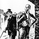1254浏览
1254
MACD Zero lag Visual inchart view.

Threshold / Settings can be changed in Format view.

Threshold to be adapted depending on timeframe.
```//@version=2
study('GreenMood inchart MACD Zero Lag', overlay=true)
// MACD VARIABLE
Range_Zerolag_BearMin = input(10)
Range_Zerolag_BearlMax = input(30)
Range_Zerolag_BullMin = input(-10)
Range_Zerolag_BulllMax = input(-30)
// MACD ZERO LAG  INPUT
source = close
fastLength = input(12, minval=1)
slowLength=input(26,minval=1)
signalLength=input(9,minval=1)
// MACD ZERO LAG  CALCULATION
// FAST LINE
ema1= ema(source, fastLength)
ema2 = ema(ema1,fastLength)
differenceFast = ema1 - ema2
zerolagEMA = ema1 + differenceFast
demaFast = (2 * ema1) - ema2
// SLOW LINE
emas1= ema(source , slowLength)
emas2 = ema(emas1 , slowLength)
differenceSlow = emas1 - emas2
zerolagslowMA = emas1 + differenceSlow
demaSlow = (2 * emas1) - emas2
//MACD LINE
ZeroLagMACD = demaFast - demaSlow
//SIGNAL LINE
emasig1 = ema(ZeroLagMACD, signalLength)
emasig2 = ema(emasig1, signalLength)
signal = (2 * emasig1) - emasig2
// MACD ZERO LAG  OUTPUT
plotredMACD = iff((crossover(signal, ZeroLagMACD) and ZeroLagMACD >Range_Zerolag_BearMin and ZeroLagMACD <Range_Zerolag_BearlMax), crossover(signal, ZeroLagMACD), na)
plotredSTRONG_MACD = iff((crossover(signal, ZeroLagMACD) and ZeroLagMACD >Range_Zerolag_BearlMax), crossover(signal, ZeroLagMACD), na)
plotgreenMACD = iff((crossover(ZeroLagMACD,signal) and ZeroLagMACD <=Range_Zerolag_BullMin and ZeroLagMACD >Range_Zerolag_BulllMax), crossover(ZeroLagMACD, signal), na)
plotgreenSTRONG_MACD = iff((crossover(ZeroLagMACD,signal) and ZeroLagMACD <Range_Zerolag_BulllMax), crossover(ZeroLagMACD, signal), na)

plotshape(plotredMACD, style=shape.labeldown, location=location.top, color=red, size=size.tiny, text="MACD Sell", textcolor=white)
plotshape(plotgreenMACD, style=shape.labelup, location=location.bottom, color=lime, size=size.tiny, text="MACD Buy", textcolor=white)
plotshape(plotgreenSTRONG_MACD, style=shape.labelup, location=location.belowbar, color=lime, size=size.tiny, text="MACD Strong Buy", textcolor=white)
plotshape(plotredSTRONG_MACD, style=shape.labeldown, location=location.abovebar, color=red, size=size.tiny, text="MACD Strong Sell", textcolor=white)```Nice work, you think you could make strategy version of this? Would be neat to backtest.TheYangGuizi
Thank you. IMHO, strategy only works if one is able to differentiate for any given timeframe what is a range from a trend. I don't believe in a strategy able to handle all kind of market condition. Regarding my own strategy, on a range market I would use a mix of MACD Zero lag, KST (I made inchart indicator for that too) and BB.